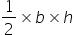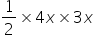Maths-
General
Easy

Question

# If the height to base ratio of a triangle ABC is 3:4 and the area is 864 square units. Determine the height and base of this triangle.Hint:

## The correct answer is: 48 units

### It is given that height to base ratio is 3 : 4⇒ Height, h = 3x and Base, b = 4xArea of triangle =864 =864  = 6x2144 = x212 = xHeight = 3x = 3(12) = 36 units and Base = 4x = 4(12) = 48 units#### With Turito Foundation.#### Get an Expert Advice From Turito.